# Plotting Correlation Matrix using Python

• Last Updated : 26 Nov, 2020

Correlation means an association, It is a measure of the extent to which two variables are related.

1. Positive Correlation: When two variables increase together and decrease together. They are positively correlated. ‘1’ is a perfect positive correlation. For example – demand and profit are positively correlated the more the demand for the product, the more profit hence positive correlation.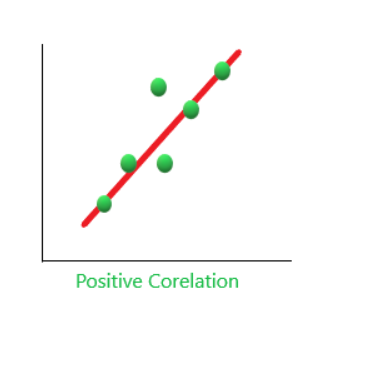2. Negative Correlation: When one variable increases and the other variable decreases together and vice-versa. They are negatively correlated. For example, If the distance between magnet increases their attraction decreases, and vice-versa. Hence, a negative correlation. ‘-1’  is no correlation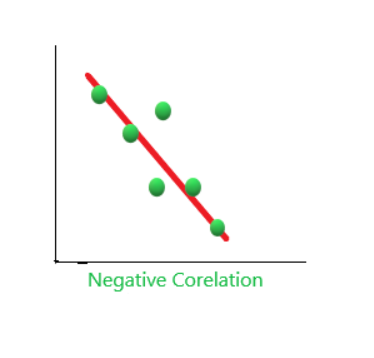3. Zero Correlation( No Correlation): When two variables don’t seem to be linked at all. ‘0’ is a perfect negative correlation. For Example, the amount of tea you take and level of intelligence.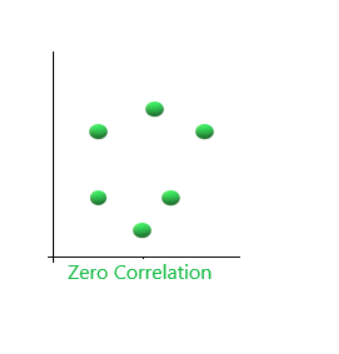## Plotting Correlation matrix using Python

Step 1: Importing the libraries.

## Python3

 `import` `sklearn``import` `numpy as np``import` `matplotlib.pyplot as plt``import` `pandas as pd`

Step 2: Finding the Correlation between two variables.

## Python3

 `y ``=` `pd.Series([``1``, ``2``, ``3``, ``4``, ``3``, ``5``, ``4``])``x ``=` `pd.Series([``1``, ``2``, ``3``, ``4``, ``5``, ``6``, ``7``])``correlation ``=` `y.corr(x)``correlation `

Output: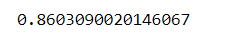Step 3: Plotting the graph. Here we are using scatter plots. A scatter plot is a diagram where each value in the data set is represented by a dot. Also, it shows a relationship between two variables.

## Python3

 `# plotting the data``plt.scatter(x, y)`` ` `# This will fit the best line into the graph``plt.plot(np.unique(x), np.poly1d(np.polyfit(x, y, ``1``))``         ``(np.unique(x)), color``=``'red'``)`

Output: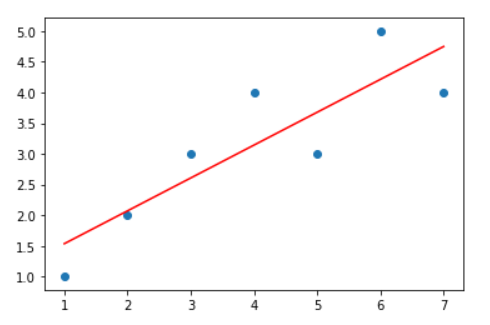Remember the points that were explained above. Observe both the images you will find similarity Also, observe the value of the correlation is near to 1, hence the positive correlation is reflected.

## Python3

 `# adds the title``plt.title(``'Correlation'``)`` ` `# plot the data``plt.scatter(x, y)`` ` `# fits the best fitting line to the data``plt.plot(np.unique(x), ``         ``np.poly1d(np.polyfit(x, y, ``1``))``         ``(np.unique(x)), color``=``'red'``)`` ` `# Labelling axes``plt.xlabel(``'x axis'``)``plt.ylabel(``'y axis'``)`

Output: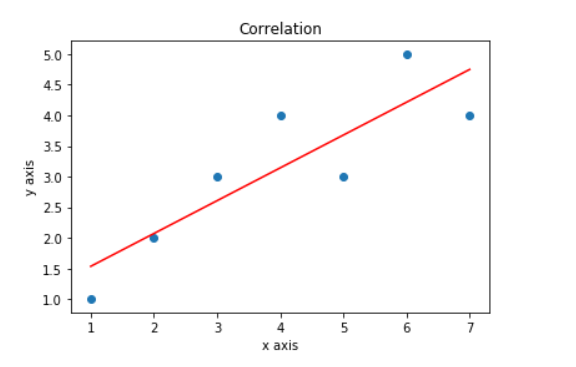## Plot using Heatmaps

There are many ways you can plot correlation matrices one efficient way is using the heatmap. It is very easy to understand the correlation using heatmaps it tells the correlation of one feature(variable) to every other feature(variable). In other words, A correlation matrix is a tabular data representing the ‘correlations’ between pairs of variables in a given data.

## Python3

 `import` `seaborn as sns`` ` `# checking correlation using heatmap``#Loading dataset``flights ``=` `sns.load_dataset(``"flights"``)`` ` `#ploting the heatmap for correlation``ax ``=` `sns.heatmap(flights.corr(), annot``=``True``)`

Output: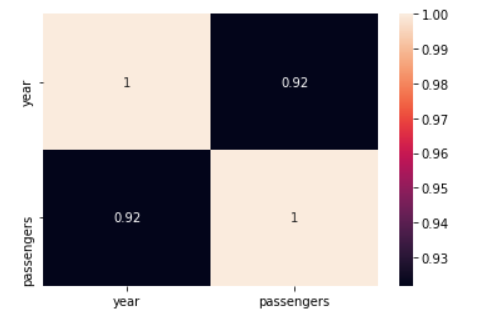My Personal Notes arrow_drop_up